#### 期刊菜单

Analysis on Obstacles Crossing of Explosion-Proof Crawler Hydraulic Support Carrier
DOI: 10.12677/ME.2019.73032, PDF, HTML, XML, 下载: 683  浏览: 2,145

Abstract: Tracked vehicle replaces traditional wheeled walking with track and wheel train. It has the ad-vantages of large carrying capacity, strong grip, low noise and turning in situ. At the same time, the use of tracked walking device also increases the contact area with the ground, which makes the bracket vehicle have good driving performance, less damage to the road surface, and strengthens its ability to cross obstacles, and strong adaptability to terrain. Therefore, the research and de-velopment of explosion-proof crawler-type hydraulic support carrier has practical significance for underground support transportation. Based on the structure design of the crawler hydraulic carrier, the dynamic model of the crawler hydraulic carrier is established. The mechanical and kinematic characteristics of the crawler hydraulic support carrier are studied by means of virtual prototype simulation and experiment. The main work of this paper is to establish the kinematics analysis model of crawler support carrier and analyze the kinematics characteristics of explosion-proof crawler hydraulic support carrier under obstacle-crossing conditions. This paper studies the movement analysis of explosion-proof crawler hydraulic support carrier in the process of obstacle crossing, and obtains the relationship between driving torque and elevation angle of explosion-proof crawler hydraulic support carrier.

1. 引言

2. 运动学模型建立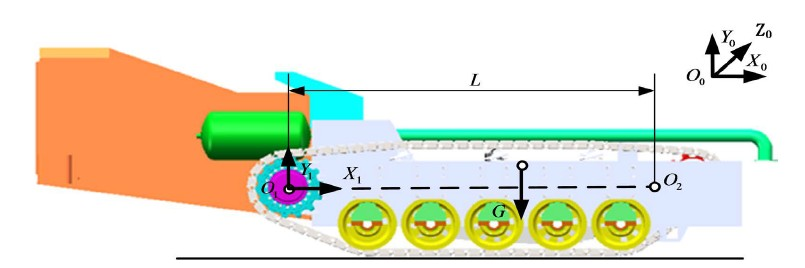Figure 1. Coordinate system of explosion-proof crawler type hydraulic bracket handling vehicle

$\begin{array}{l}RPY\left(\beta ,\gamma ,\alpha \right)=Rot\left(y,\gamma \right)Rot\left(x,\beta \right)\\ =\left[\begin{array}{ccc}\mathrm{cos}\alpha \mathrm{cos}\gamma & -\mathrm{sin}\alpha \mathrm{cos}\beta +\mathrm{cos}\alpha \mathrm{sin}\gamma \mathrm{sin}\beta & \mathrm{sin}\alpha \mathrm{sin}\beta +\mathrm{cos}\alpha \mathrm{sin}\gamma \mathrm{cos}\beta \\ \mathrm{sin}\alpha \mathrm{cos}\gamma & \mathrm{cos}\alpha \mathrm{cos}\beta +\mathrm{sin}\alpha \mathrm{sin}\gamma \mathrm{sin}\beta & -\mathrm{cos}\alpha \mathrm{sin}\beta +\mathrm{sin}\alpha \mathrm{sin}\gamma \mathrm{cos}\beta \\ -\mathrm{sin}\gamma & \mathrm{cos}\mathrm{sin}\beta & \mathrm{cos}\gamma \mathrm{cos}\beta \end{array}\right]\end{array}$ (2.1)

${}^{0}T=\left[\begin{array}{cccc}\mathrm{cos}\alpha \mathrm{cos}\gamma & -\mathrm{sin}\alpha \mathrm{cos}\beta +\mathrm{cos}\alpha \mathrm{sin}\gamma \mathrm{sin}\beta & \mathrm{sin}\alpha \mathrm{sin}\beta +\mathrm{cos}\alpha \mathrm{sin}\gamma \mathrm{cos}\beta & a\\ \mathrm{sin}\alpha \mathrm{cos}\gamma & \mathrm{cos}\alpha \mathrm{cos}\beta +\mathrm{sin}\alpha \mathrm{sin}\gamma \mathrm{sin}\beta & -\mathrm{cos}\alpha \mathrm{sin}\beta +\mathrm{sin}\alpha \mathrm{sin}\gamma \mathrm{cos}\beta & b\\ -\mathrm{sin}\gamma & \mathrm{cos}\gamma \mathrm{sin}\beta & \mathrm{cos}\gamma \mathrm{cos}\beta & c\\ 0& 0& 0& 0\end{array}\right]$ (2.2)

$\gamma$ ——机身绕y轴旋转角；

$\alpha$ ——机身绕z轴旋转角；

$\left(a,b,c\right)$ ——坐标系 ${O}_{1}\text{-}{X}_{1}{Y}_{1}{Z}_{1}$ 的原点在 ${O}_{0}\text{-}{X}_{0}{Y}_{0}{Z}_{0}$ 中的坐标。

${}^{0}p={}^{0}T{}^{1}p=\left[\begin{array}{c}\mathrm{cos}\alpha \mathrm{cos}\gamma {L}_{x}+\left(-\mathrm{sin}\alpha \mathrm{cos}\beta +\mathrm{cos}\alpha \mathrm{sin}\gamma \mathrm{sin}\beta \right){L}_{y}+a\\ \mathrm{sin}\alpha \mathrm{cos}\gamma {L}_{x}+\left(\mathrm{cos}\alpha \mathrm{cos}\beta +\mathrm{sin}\alpha \mathrm{sin}\gamma \mathrm{sin}\beta \right){L}_{y}+b\\ -\mathrm{sin}\gamma {L}_{x}+\mathrm{cos}\gamma \mathrm{sin}\beta {L}_{y}+c\\ 1\end{array}\right]$ (2.3)

${}^{0}p=\left[\begin{array}{c}\mathrm{cos}\alpha {L}_{x}-\mathrm{sin}\alpha {L}_{y}+a\\ \mathrm{sin}\alpha {L}_{x}+\mathrm{cos}\alpha {L}_{y}+b\\ c\\ 1\end{array}\right]$ (2.4)

3. 转向运动关系Figure 2. Schematic diagram of the positional relationship of the steering process

$\omega \left(R+{\left(-1\right)}^{i}B/2\right)+\omega {A}_{i}={v}_{i},\text{\hspace{0.17em}}i=1,2$ (3.1)

$\omega$ ——车辆转动的角速度；

${R}_{1}$ ——理论转向半径。

${A}_{i}={v}_{ayi}/\omega ,\text{\hspace{0.17em}}i=1,2$ (3.2)

$\left\{\begin{array}{l}{a}_{i}={A}_{i}/\left(L/2\right)\\ d=D/\left(L/2\right)\end{array}$ (3.3)

$\rho =\frac{1}{2}\frac{\left(1+\lambda {a}_{1}\right)+{K}_{v}\left(1+\lambda {a}_{2}\right)}{1-{K}_{v}}={f}_{\rho }{\rho }_{t}$ (3.4)

${\rho }_{t}$ ——不考虑履带滑移情况的相对转弯半径， ${\rho }_{t}=\frac{1}{2}\left(\frac{1+{K}_{v}}{1-{K}_{v}}\right)$

$\lambda$ ——履带贴地长度L和履带中心距离B之比， $\lambda =L/B$

fρ——履带滑移时，相对转弯半径和不考虑履带滑移的相对转弯半径比例系数，即转弯半径修正系数，表达式为：

${f}_{\rho }=\frac{\left(1+\lambda {a}_{1}\right)+{K}_{v}\left(1+\lambda {a}_{2}\right)}{1+{K}_{v}}$ (3.5)

$\omega =\frac{{v}_{2}-{v}_{1}}{{B}^{\prime }+{A}_{2}+{A}_{1}}={f}_{\omega }{\omega }_{t}$ (3.6)

${\omega }_{t}$ ——不考虑履带滑移条件下的角速度， ${\omega }_{t}=\left({v}_{2}-{v}_{1}\right)/{B}^{\prime }$

${f}_{\omega }$ ——考虑履带滑移时相对转弯半径和不考虑履带滑移的角速度比例系数，即转弯半径修正系数，表达式为：

${f}_{\omega }=\frac{1}{1+\lambda /2\left({a}_{2}-{a}_{1}\right)}$ (3.7)

$\left\{\begin{array}{l}{v}_{r}=\left(\rho -\frac{1}{2}B\right)\omega \\ {v}_{l}=\left(\rho +\frac{1}{2}B\right)\omega \end{array}$ (3.8)

$\left\{\begin{array}{l}{v}_{r}=R{\omega }_{r}\\ {v}_{l}=R{\omega }_{l}\end{array}$ (3.9)

$\omega =\frac{R{\omega }_{r}}{\rho -\frac{1}{2}B}=\frac{R{\omega }_{L}}{\rho +\frac{1}{2}B}$ (3.10)

$\omega =\frac{R\left({\omega }_{l}-{\omega }_{r}\right)}{B}$ (3.11)

$\rho =\frac{\frac{1}{2}B\left({\omega }_{l}+{\omega }_{r}\right)}{{\omega }_{l}-{\omega }_{r}}=\frac{1}{2}B\left(1+\frac{2}{{\omega }_{l}/{\omega }_{r}-1}\right)$ (3.12)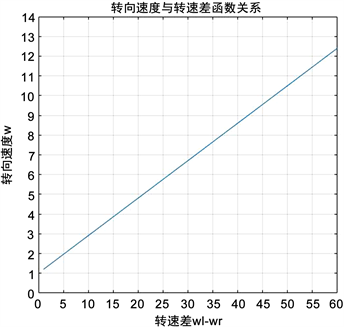(a) 转向速度与转速差函数关系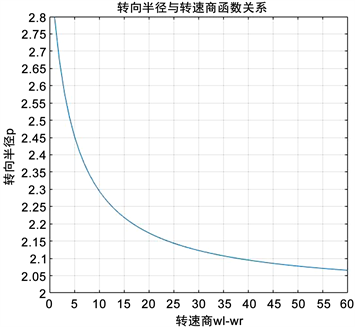(b) 转向半径与转速商函数关系

Figure 3. Relationship between swing speed and radius of gyration

4. 防爆履带式液压支架搬运车越障运动分析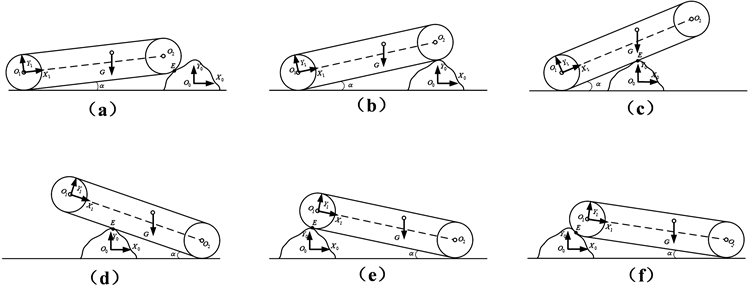Figure 4. Obstacle-crossing process of explosion-proof crawler type hydraulic bracket handling vehicle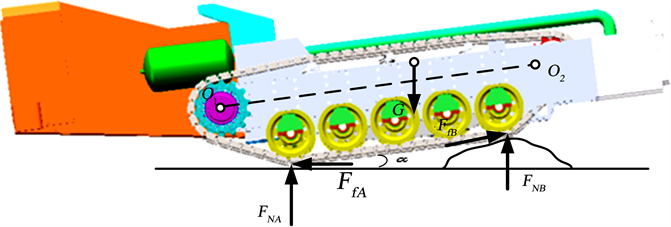Figure 5. Stress of explosion-proof crawler type hydraulic bracket handling vehicle

$\left\{\begin{array}{l}\left\{T+G\left({L}_{x}\mathrm{cos}\alpha -{L}_{y}\mathrm{sin}\alpha \right)+{F}_{fB}\mathrm{cos}\alpha \left(R+L\mathrm{sin}\alpha -R\right)\\ -\left({F}_{fB}\mathrm{sin}\alpha +{F}_{NB}\right){L}_{1}\mathrm{cos}\alpha =0\\ {F}_{fB}=\mu {F}_{NB}\end{array}$ (4.1)

${F}_{NA},{F}_{NB}$ ——搬运车受到地面的支撑力；

G——搬运车本体结构重力；

T——输出转矩；

$\mu$ ——摩擦系数，取 $\mu =0.5$

$\alpha$ ——搬运车仰角，其中规定防爆履带式液压支架搬运车逆时针旋转为正。

$T=\left({F}_{fB}\mathrm{sin}\alpha +{F}_{NB}\right){L}_{1}\mathrm{cos}\alpha -G\left({L}_{x}\mathrm{cos}\alpha -{L}_{y}\mathrm{sin}\alpha \right)-{F}_{fB}\mathrm{cos}\alpha L\mathrm{sin}\alpha$ (4.2)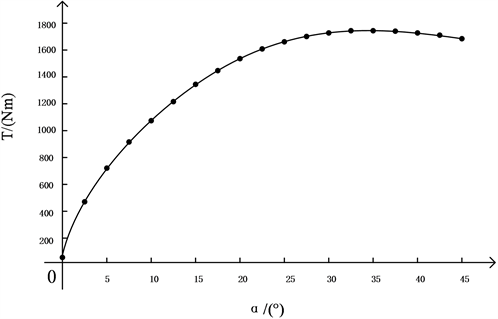Figure 6. Curve of driving torque variation with elevation angle of bracket carrier

5. 结论

  姜泽. 煤矿综合机械化采煤工艺[J]. 矿业装备, 2018(4): 72-73.  蔡毅. 液压支架分析优化技术研究[D]: [硕士学位论文]. 武汉: 华中科技大学, 2012.  李博. 通用型支架搬运车方案设计及液压同步提升系统研究[D]: [硕士学位论文]. 太原: 中北大学, 2016.  张波. WC50Y大坡度支架搬运车的研制及应用[J]. 陕西煤炭, 2016, 35(6): 79-82.  张国栋. 煤矿井下支架搬运车的发展趋势及合理选用[J]. 煤矿机械, 2012, 33(8): 196-197.  姚志功. 影响煤矿支架搬运车轮胎损耗的因素分析及预防措施[J]. 煤炭工程, 2016, 48(7): 132-133.  姚志功. 煤矿铲板式支架搬运车超载使用的危害及对策[J]. 中国煤炭, 2015, 41(5): 91-93.  姚禹. 基于履带-地面耦合系统的低速履带车辆通过性研究[D]: [博士学位论文]. 长春: 吉林大学, 2016.  胡庆龙. 关节履带式移动机器人控制系统设计及爬楼梯分析[D]: [硕士学位论文]. 哈尔滨: 哈尔滨工业大学, 2010.  王伟东. 面向复杂地面环境的作业型履带式移动机器人研究[D]: [博士学位论文]. 哈尔滨: 哈尔滨工业大学, 2009.